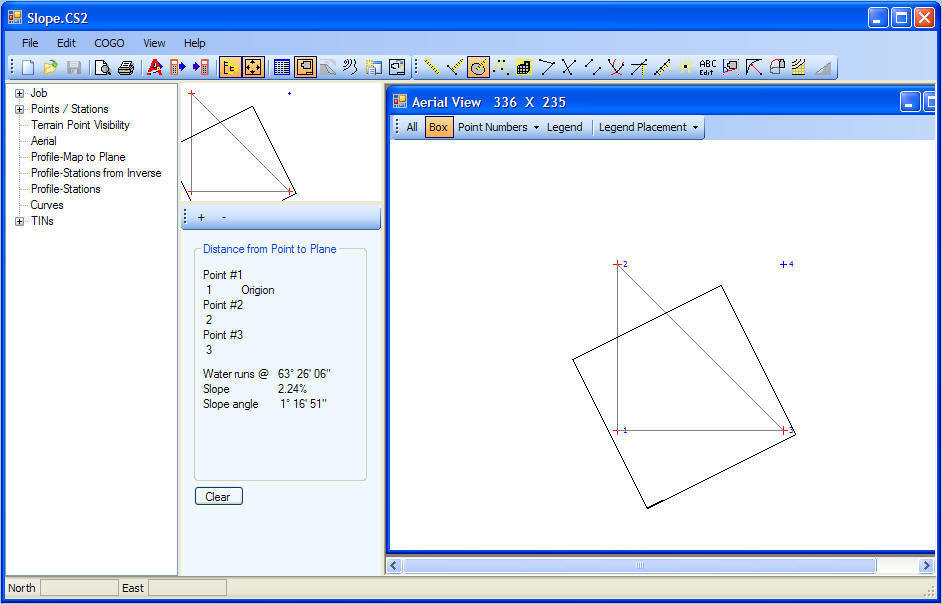Laser Setup - Convert dual slopes to single slopeSlope from point 1 to point 2 is -1%
Slope from point 1 to point 3 is -2%
These 2 slopes are the same as -2.24% at an azimuth of 63 26' 06"
This is calculated with Point to Plane - Choose the 3 points which represent the plane you want to grade - the slope and direction will be solvedback